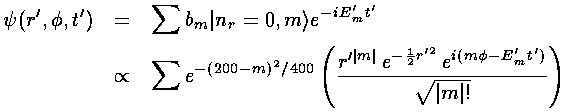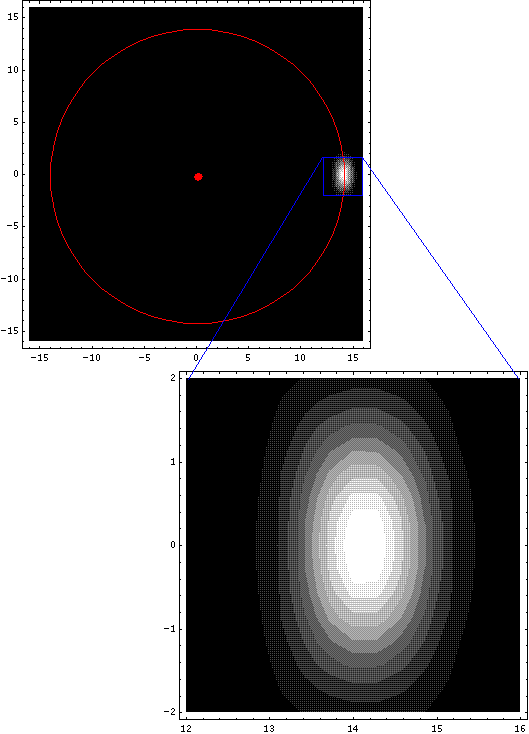## SHM p.11

### Seeing QM Oscillation: 2-d

It is quite easy to produce visible x-y oscillation: just find the sum of y wavefunctions that oscillates in y (working exactly as in the 1-d case) and find the sum of x wavefunctions that oscillates in x and multiply them together. Most of the probability will be in a lump at a particular (moving) (x,y).

To build up an orbiting probability packet, we must use wavefunctions with minimal inward/outward motion (i.e., nr=0) and large angular momentum (i.e., large positive m). We will use as our model wavefunction a function that is localized inbut with lots of oscillation in, i.e., exp[-1002+200i]). Our |nr=0, m> wavefunctions do, of course, depend on r: basically restricting the wavefunction to r near m½, i.e., the bottom of the effective potential. But for small variations in m, this will be much the same r. Thus we focus on localizing in. We start by straightforward Fourier expansion of our model wavefunction in terms of exp(im), thebehavior of the |nr=0, m> wavefunctions.Using these same bm to expand our wavefunction should achieve similar localization inwhile localization in r' is automatic for large m, nr=0 wavefunctions. In practice one can't sum over an infinity of m, but the equation for bm shows that bm is large only for m near 200 (so we restrict our sum to between 180 and 220).Below is displayed the resulting lump of probability for t'=0.We need not display similar plots for other times because the equation forshows that plot for any other time are identical to the above except rotated by 2t' radians. Thus the lump will make one complete revolution in a period of